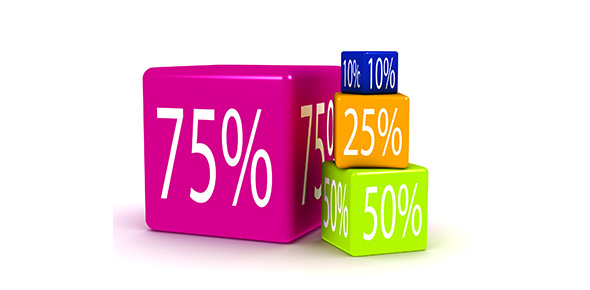# Math Quiz On Percentage! Test

14 Questions | Total Attempts: 35Settings.

Related Topics
• 1.
What is 8/9 as a decimal?
• A.

.89

• B.

.87

• C.

0.87

• D.

0.89

• 2.
What is 5/8 as a decimal?
• A.

0.63

• B.

0.78

• C.

0.56

• D.

0.64

• 3.
What is 2/3 as a decimal?
• A.

0.67

• B.

0.76

• C.

0.72

• D.

0.89

• 4.
What is 3/4 as a decimal?
• A.

.75

• B.

0.76

• C.

0.75

• D.

.74

• 5.
What is 0.98234 as a percent?
• A.

98%

• B.

97%

• C.

92%

• D.

982%

• 6.
What is 0.34  as a percent/
• A.

340%

• B.

034%

• C.

34%

• D.

3400%

• 7.
What is 0.0056 as a percent?
• A.

560%

• B.

5.6%

• C.

0.6%

• D.

56%

• 8.
What is 7.8 as a Percent?
• A.

780%

• B.

0.078%

• C.

78%

• D.

None of the above

• 9.
What is 2.33 as a percent?
• A.

23.3%

• B.

233%

• C.

0.00233

• D.

None of the above

• 10.
What is 6/17 as a percent?
• A.

60%

• B.

67%

• C.

35%

• D.

32%

• 11.
What is 30% of 120?
• A.

36%

• B.

3.6%

• C.

63%

• D.

None of the above

• 12.
9 is what percent of 45?
• A.

25%

• B.

20%

• C.

65%

• D.

None of the above

• 13.
18 is 9% of what number?
• A.

201%

• B.

200%

• C.

102%

• D.

None of the above

• 14.
11/100 is what percent?
• A.

0.11%

• B.

1.1%

• C.

11%

• D.

None of the above